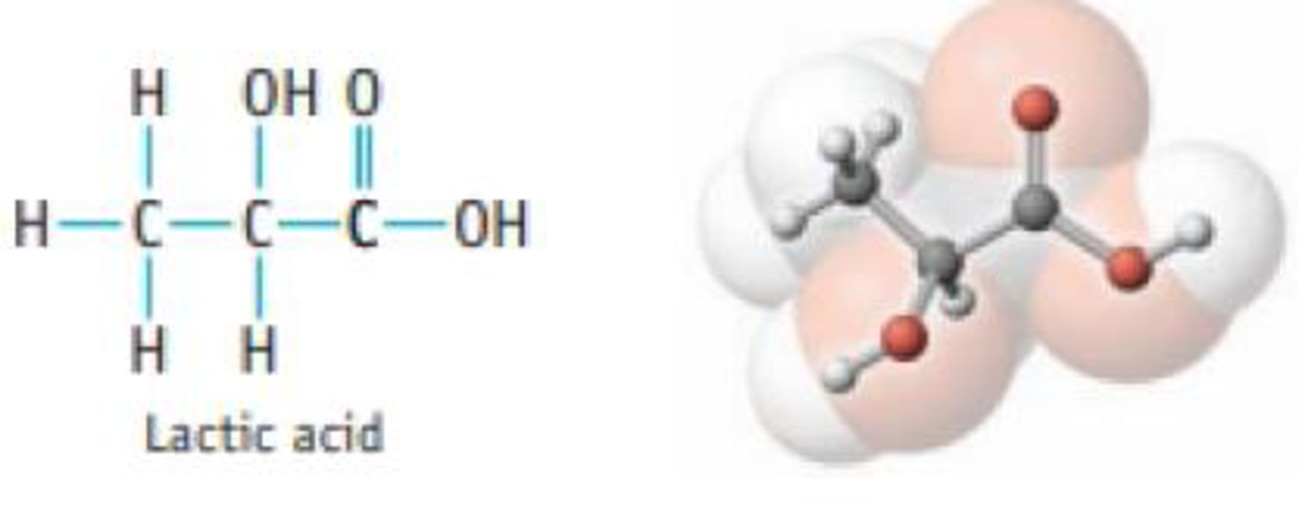Chapter 3, Problem 95SCQ

Chapter
Section
Textbook Problem

Most naturally occurring acids are weak acids. Lactic acid is one example. CH 3 CH(OH)CO 2 H(s) + H 2 O( l ) ⇄ H 3 O + (aq) +    CH 3 CH(OH)CO 2 − (aq) If you place some lactic acid in water, it will ionize to a small extent, and an equilibrium will be established. Suggest some experiments to prow that this is a weak acid and that the establishment of equilibrium is a reversible process.Interpretation Introduction

Interpretation:

The experiments that proves Lactic acid is weak acid and equilibrium is reversible should be explained.

Concept introduction:

Acids can donate the H+ ion, base can accept the H+ ion.  Strong acids ionize in water very easily, weak acid will ionize very slowly.  Strong acid is one that completely ionizes (dissociates) in a solution (provided there is sufficient solvent).  In water, acid HA dissolves and gives H+ (as hydronium ion H3O+ ion).  A strong electrolyte that’s an acid or a base is also a strong acid or a strong base.  A weak electrolyte that’s an acid or a base is also a weak acid or a weak base.

Explanation

Weak electrolyte test:

The conductivity of a solution lactic acid is significantly less because, conductivity of lactic acid (weak acid) is less when compare to conductivity of a solution of strong acid.

Reversible reaction:

Lactic acid which undergoes dissociation, which produce lactate ion and H3O+ ion, which is shown below,

CH3CH(OH)COOH(aq)+H2O(l)

Still sussing out bartleby?

Check out a sample textbook solution.

See a sample solution

The Solution to Your Study Problems

Bartleby provides explanations to thousands of textbook problems written by our experts, many with advanced degrees!

Get Started